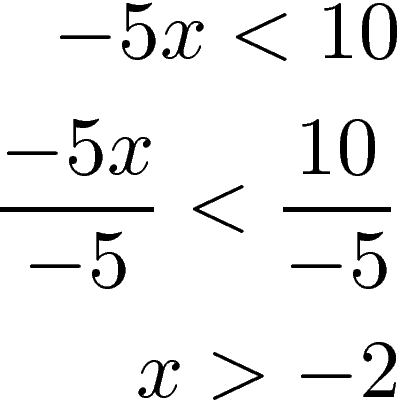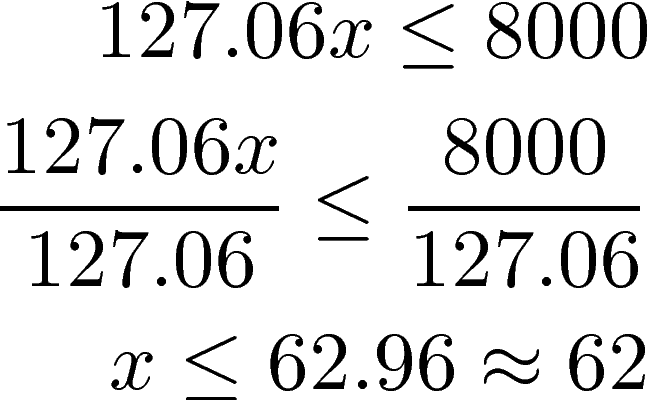# Solving Inequalities

Inequalities are equations that use symbols related to less than, greater than, etc. This allows for the solution to be a range of values rather than only one specific one as in many standard equations where you solve for x.Unique Property of Inequalities

The rules for solving inequalities are mostly the same as for solving a regular equation with one exception. If you multiply or divide both sides of an inequality by a negative number you need to flip the inequality sign.  Below is an example of the sign flippingIf you look at the final answer you can see that the x must be greater than -2. This makes sense as -5 * -2 would come to 10 which is not less than 10. Naturally,  any number that is larger than -2 would only be worst. Below is a word problem that employs an inequality.

Single Inequality

You have \$8,000 to buy math textbooks for your classroom. Each math book cost \$127.06. What is the maximum number of math books you can buy?

In the problem above, the keyword is maximum. In other words, there is a range of potential answers from 1 book to whatever the max is. This indicates that this problem is an inequality. Therefore,

• Let 127.06 be the price of a math book
• x the number of math books we can buy
• < use less than because we do not want to exceed our budget of 8000

Below is the solution to the problem.You can buy up to 62 books and be less than or equal to 8000. We round down to 62 because we must stay under \$8,000 in spending.

Below is another example but slightly more complex as it contains additional information.

Complex Single Inequality

You are planning a three-day camping trip for your students. Currently, there is \$420 of money available. The students can earn \$22.50 per hour through tutoring. The trip will cost \$525 for transportation,  \$390 for food, and \$47.50 per night for the campground.  How many hours do the students need to tutor in order to have enough money for the trip?

This problem has three pieces of information on the left of the inequality

• Transportation (525)
• Food (390)
• campground per night (47.5 * 3)

The information to the right is the following

• The money available (420)
• The earning rate per hour (22.50)
• The variable for the hours to tutor (x)

We use the less than or equal to inequality <

Below is the solutionThe students need to tutor for at least 28hours and 20 minutes in order to meet the expenses for the trip.

Conclusion

Inequalities are another useful tool taught in algebra. The applications are limitless. The key to appreciating inequalities is being able to determine when they can be used to solve real-world problems.